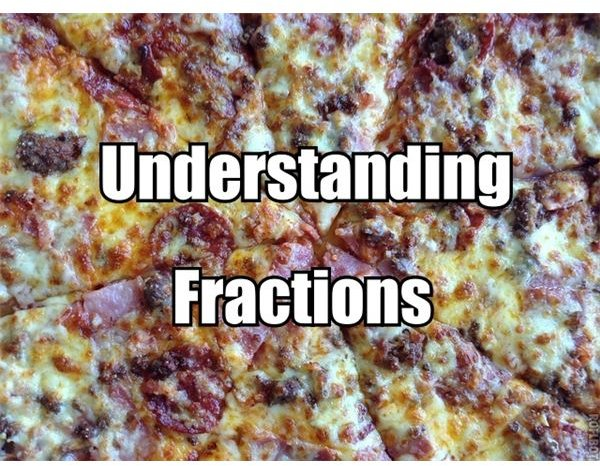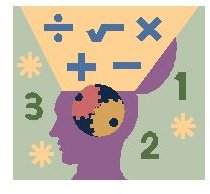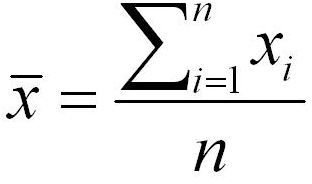# Help with Math Homework

Get the math help you need at Bright Hub Education. You’ll find homework help and study tips for all grade levels of K – 12, from basic addition and subtraction guides to advanced algebra and calculus concepts. Whether you are a grade school student looking for multiplication and division techniques, a middle school student struggling with early algebraic concepts, or a high school student studying trigonometry and advanced calculus in preparation for AP or college level math, you’ll find clear, concise and helpful guidance on the math concepts and problems you’ve been struggling with.Sometimes math seems challenging until you can see and make sense of what you are doing. You work with fractions all the time but you may not realize it. We will go over some principles—facts that are always true—about fractions, and the next time you work with them, you will recognize and know it.

## Step-By-Step Guide to Long Division: Remember all the Steps

One of the challenges of long division problems is remembering the many different steps. Learn some little tricks to help you remember all the steps in this study guide.

## Learning About Multiplication and Division: Practice Quiz

Looking to improve your understandng of multipication and division? Try taking our quiz to see how much you know.Helping your child with fractions homework is a click away. Browse throughout this table of contents to understand and apply strategies for assisting your child, with or without special needs, with fractions homework. Be prepared to take the stress out of homework sessions involving fractions.

## Test Your Trigonometry Knowledge. Do You Know a Sine from a Cosine or a Tangent?

Trigonometry doesn’t have to be a challenge. Take this quiz to determine if you know your trig functions. You’ll know the difference between a sine, cosine and tangent in no time at all.

## An Elementary Math Operation Cheat Sheet With Problem Examples For Each Operation

This cheat sheet for elementary math will help you to remember the basic rules. Use this as a review or as a study sheet to stay current in your studies. Use this sheet to practice solving all types of math problems and never worry about forgetting your basic math rules again.## Math Homework Help – How to Calculate Weighted Average – Tips and Formula for Calculating Weighted Averages

Many students ask how to calculate weighted averages. Understanding the technique is quite essential for solving math problems at almost any level. In this article, we will present to formula for calculating weighted averages and offer some quick calculation tips.

## Practice Math Ratios at Home With Games & Practice Problems

This article provides the reader with fun and creative ways to practice learning about ratios while at home. You will also find online resources with free math ratio games, instructions and quizzes as well as videos illustrating and teaching the concept of ratios.## Find the Quotient of a Division Word Problem: Know How to Identify the Dividend and the Divisor

Learn how to find the quotient of a division word problem by following the tips and techniques provided by this article. Read the explanations for basic concepts and components to serve as a guide for formulating the equation – then download the drill practice calculations.

## Dividing Mixed Fractions in Four Easy Steps

Dividing mixed fractions is easier than it sounds. Just follow this step-by-step guide to divide any two mixed fractions your teacher can throw at you!

## Solving Square Roots: Simplification, Addition, Subtraction, Multiplication and Division

If you’re having trouble, remember most square root problems are based on either simplification, addition, subtraction, multiplication, division or a mixture. Read on for simple instructions, and you’ll never be afraid of square roots again!

## Adding and Subtracting Three-Digit Numbers: How To and Examples

Adding and subtracting three-digit numbers is very similar to adding and subtracting two-digit numbers. You just have an extra place, the hundreds place, to work with. Here are the steps for both types of problems and some online resources where you can practice.

## A Study Guide for Subtracting Mixed Fractions With Regrouping with Examples

Mixed fractions, also known as mixed numbers, consist of a whole number and a fraction. When you subtract two mixed numbers, you may have to regroup to work the problem. This study guide will help you work these types of problems.